# The systems of sequences and the transition formulas

## 28.1 The system of sequences

The common situation, an operator Yt of the complicated algorithm (describing, for example, the functioning of the automated management system) depends on finite subset of logic conditions {xi1,…,xir} out of the total logic conditions set X.

In this connection, it is convenient to use the specialized language for algorithms determining.

An elementary sequence determines the condition to execute the following operator Yj after the operator Yi. A sequence is represented in a form (28.1):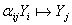(28.1)

The symbol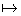means “if then”. The formula (28.1) is to be read in such the way: the operator Yj will be executed after the operator Yi if  the condition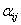holds true.

If the operator Yj has many operators- predecessors, the sequence is represented in the generalized form the system of sequences: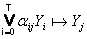, j=1,…,T.                                  (28.2)

An example of algorithm representation in a form of the system of sequences:(28.3)

It is easy to obtain the MSA right out of the system of sequences (the table 28.1).

The sequence representation of an algorithm has advantage of compactness. Further, having obtained the MSA, the system of transition formulas may be written down.

Table 28.1

 MSA Y1 Y2 Y3 Y4 YT Y0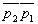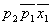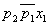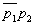Y1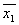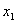Y2 1 Y3 1 Y4 1

### Информация о работе

Тип:
Лекции, учебные пособия
Размер файла:
78 Kb
Скачали:
1